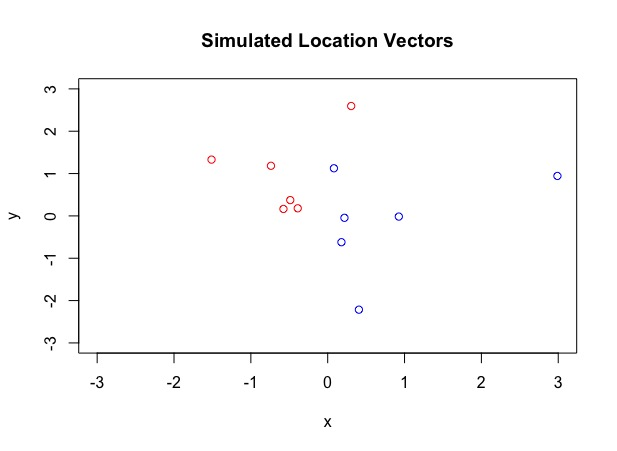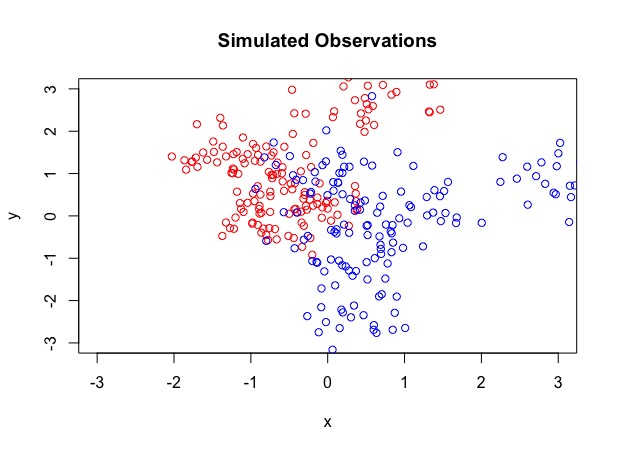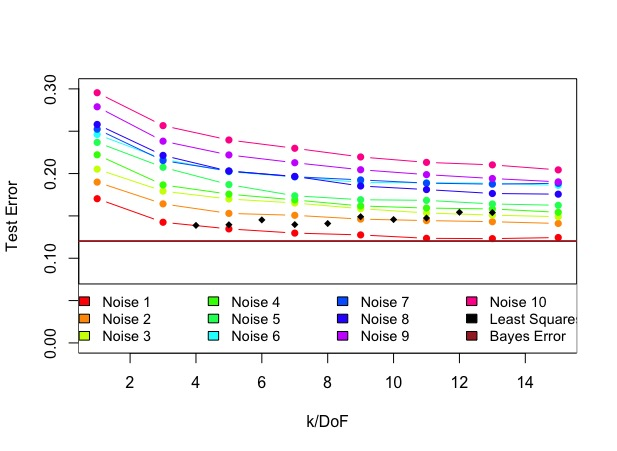# Linear Regression and the KNN

This was an homework problem in STATS315A Applied Modern Statistics: Learning at Stanford and I thought it is worth sharing. It runs a simulation to compare KNN and linear regression in terms of their performance as a classifier, in the presence of an increasing number of noise variables.

## Model

We have a binary response variable $Y$, which takes value ${0,1}$. The feautre variable $X$ is in $\mathcal{R}^{2 + k}$, of which 2 are the true features and the rest $k$ are noise features. The model used to simulate the data is a Gaussian Mixture. First we generate 6 location vectors $m_{k}$ in $\mathcal{R}^{2}$ from a bivariate Gaussian $N[(1,0)^{T}, \boldsymbol{I}]$ with $Y = 1$ and 6 location vectors from $N[(0,1)^{T}, \boldsymbol{I}]$ with $Y = 0$. To simulate $n$ observations from each class, we picked an location vector $m_k$ with a probaility of $1/6$ and then generate one observation from $N[m_k, \boldsymbol{I}/5]$.

## Data Simulation## Bayes Clasifier

Given that we know the underlyign model, we can compute the Bayes Classifier $$\hat{Y}(x) = \text{argmax}_Y(Pr(Y|X=x))$$ In our case, we can find the closest location vector to an observation and assign the observation to its class.

We adds up to $K$ noise features to the training data, drawing each noise observations from the uniform normal distribution $N(0,1)$

## ResultsOverall, the test error of KNN decreases as $k$ increases, no matter how many noise parameters there are The test error of KNN generally increases significantly as the number of noise parameters increases, while the test error of least squares stays at about the same level. This shows that the KNN is more susceptible to high noise due to its flexiblity. The least squares is more rigid and is less affected by the noise. KNN overperforms the least squares when the noise-to-signal ratio is low and underperforms the least squares when the noise-to-signal ratio is high.